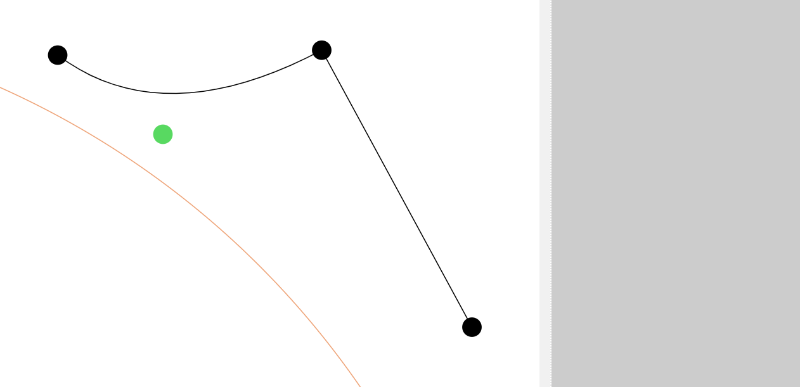This tool creates a corner by trimming or extending two lines or curves that can cross. This will not work if the selected points have more than one line or curve attached.

Important!

In order for this tool to work properly, the two items (lines or curves) must cross using their current vectors.

## Trimming

This will trim two lines or curves that cross. Select the two end points that you want trimmed off.

Here are two lines.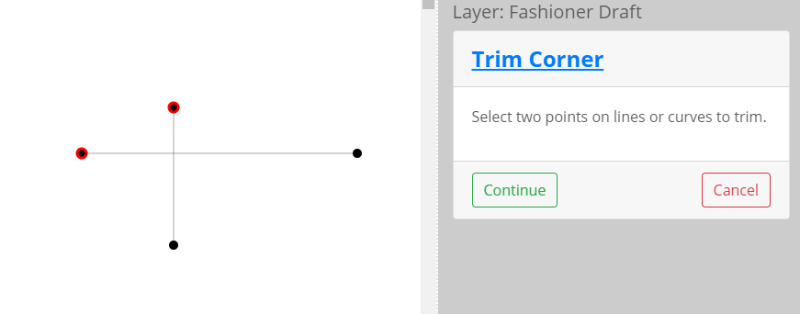Here are a line and curve.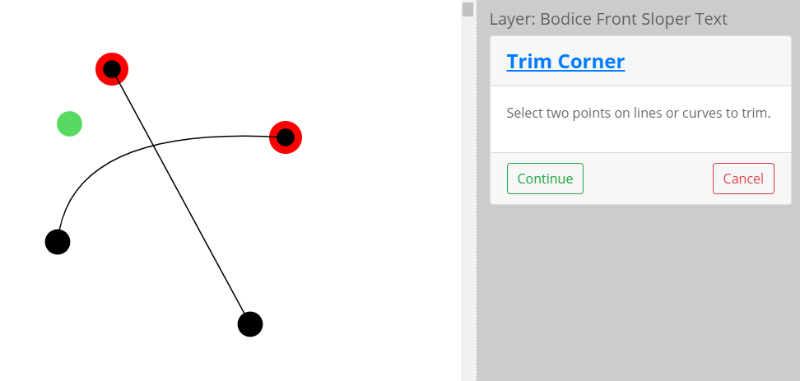The selected items will be shortened and now share the same point. First is the two lines.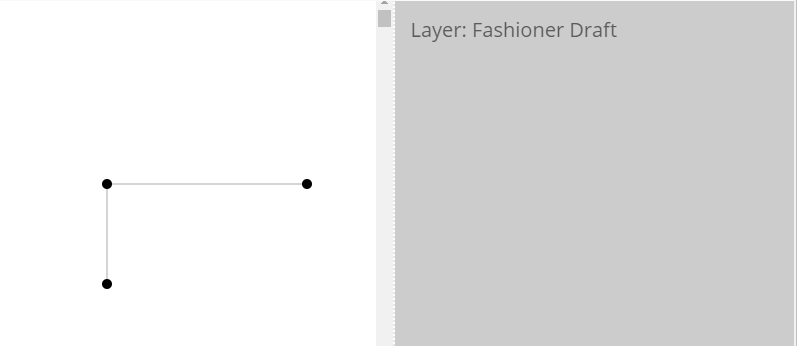Here is the line and curve. Notice that the shape of the curve has been distorted. If you need to preserve that shape, use the Curve to Line tool before trimming.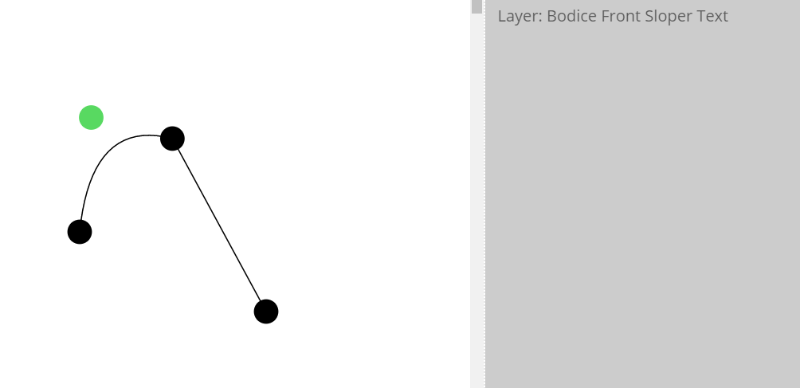## Extending

This will extend two lines or curves to meet at a point. Here is a two line selection.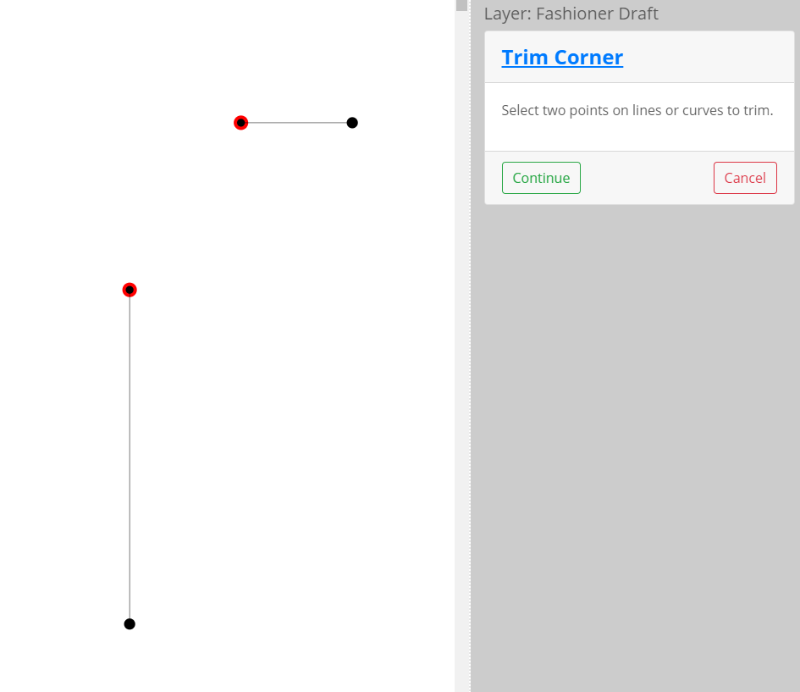Here are a line and curve selection.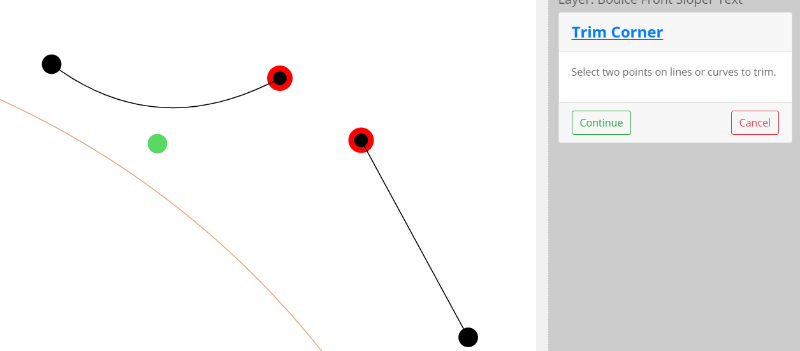Here is the result for the lines extension.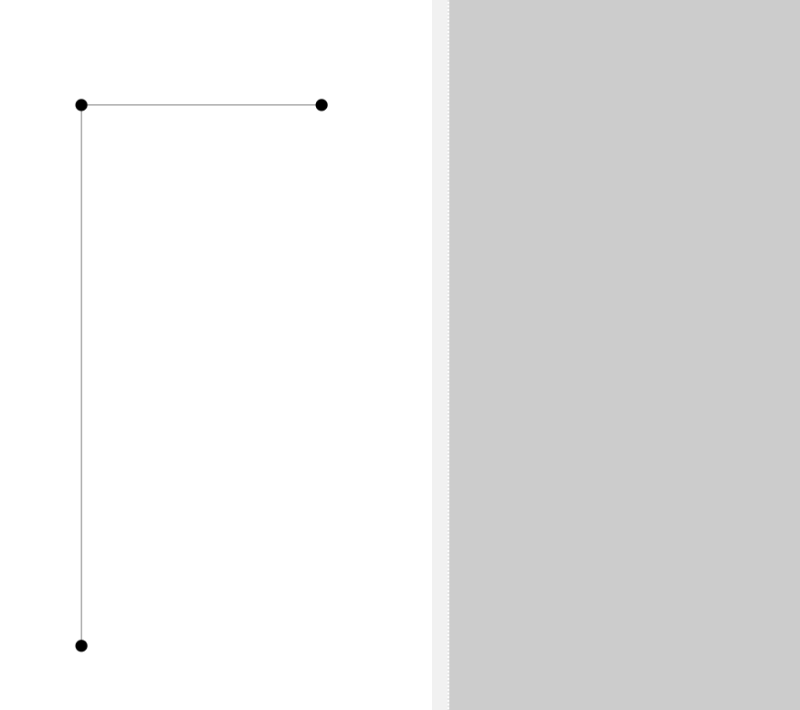Here is the result of the line and curve extension. Again, the shape of the curve has been changed.